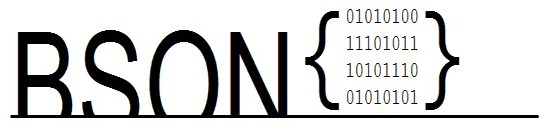# Introduction to BSON

## What is BSON

A single entity in BSON is called as a document. A document comprised of zero or more key/value pairs(like associative arrays) in binary format.## Datatypes

BSON supports the following basic datatypes :

• byte : 1 byte, i.e. 8 bits.
• int32 : 4 bytes i.e. 32-bit signed integer.
• int64 : 8 bytes i.e. 64-bit signed integer.
• double : 8 bytes i.e. 64-bit IEEE 754 floating point

The following table describes the rest specifications of a BSON document :

Grammar Description
document ::=int32 e_list "\x00" BSON Document : init32 refers to the total number of bytes of the document.
e_list ::= element e_list | "" Sequence of elements.
element ::= "\x01" e_name double Floating point.
element ::= "\x02" e_name string UTF-8 string.
element ::= "\x03" e_name document Embedded document.
element ::= "\x04" e_name document Array.For example ['x','y'] will be represented as {'0':'x','1':'y'}
element ::= "\x05" e_name binary Binary data
element ::= "\x06" e_name Undefined(this has been deprecated)
element ::= "\x07" e_name (byte*12) ObjectId
element ::= "\x08" e_name "\x00" Boolean "false"
element ::= "\x08" e_name "\x01" Boolean "true"
element ::= "\x09" e_name int64 UTC milliseconds in UNIX epoch
element ::= "\x0A" e_name Null value
element ::= "\x0B" e_name cstring cstring Regular expression
element ::= "\x0C" e_name string (byte*12) DB Pointer. This has been deprecated.
element ::= "\x0D" e_name string JavaScript Code.
element ::= "\x0E" e_name string Symbol.
element ::= "\x0F" e_name code_w_s JavaScript code w/ scope.
element ::= "\x10" e_name int32 32-bit Integer.
element ::= "\x11" e_name int64 Timestamp.
element ::= "\x12" e_name int64 64-bit integer.
element ::= "\xFF" e_name Min key.
element ::= "\x7F" e_name Max key.
e_name ::= cstring Key name.
string ::= int32 (byte*) "\x00" String.
cstring ::= (byte*) "\x00" CString.
binary ::= int32 subtype (byte*) Binary.
subtype ::= "\x00" Binary / Generic.
subtype ::= "\x01" Function.
subtype ::= "\x02" Old generic subtype.
subtype ::= "\x03" UUID.
subtype ::= "\x05" MD5.
subtype ::= "\x80" User defined.
code_w_s ::= int32 string document Code w/ scope.

## Implementation

There are several BSON libraries available in various languages like - ActionScript,C,C# / .Net, C++ or stand-alone C++, Delphi, Erlang, Factor, Fantom, Go, Haskell, Java, J2ME (work in progress), Lisp, Perl, PHP, Python — with optional C extension, Python 3, Ruby — with optional C extension, Standard ML (SML)

MongoDB, which is a NoSQL database, stores data in BSON format.

## Comparison between BSON and JSON

For the sake of faster navigation within BSON, it adds some extra fields that JSON, like length prefixes. Because of this, though it is designed to be taken less space that JSON, in practice, sometimes JSON takes less space.

BSON is faster than JSON when it comes to encoding and decoding.

Previous: Working with JSONPath and PHP
Next: JSONP Tutorial

﻿